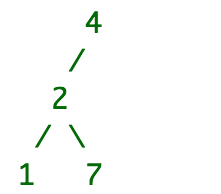# Can someone help me with this js problem?

### Tree Constructor

Have the function TreeConstructor( strArr ) take the array of strings stored in strArr , which will contain pairs of integers in the following format: (i1,i2) , where i1 represents a child node in a tree and the second integer i2 signifies that it is the parent of i1 . For example: if strArr is [“(1,2)”, “(2,4)”, “(7,2)”], then this forms the following tree:which you can see forms a proper binary tree. Your program should, in this case, return the string true because a valid binary tree can be formed. If a proper binary tree cannot be formed with the integer pairs, then return the string false . All of the integers within the tree will be unique, which means there can only be one node in the tree with the given integer value.

#### Examples

Input: [“(1,2)”, “(2,4)”, “(5,7)”, “(7,2)”, “(9,5)”]
Output: true

Input: [“(1,2)”, “(3,2)”, “(2,12)”, “(5,2)”]
Output: false

Hi there and welcome.

It looks like you are working through some learning material. Can you please let us know what you have attempted already.

Here is also some useful advice about how to post code from the Forum Posting Basics thread.

This is my attempt trying to solve the problem .

``````function TreeConstructor(strArr) {
var arr = [ ], counts= {};
for (i=0;i < strArr.length;i++){
arr.push(strArr[i].match(/[0-9]/g));
arr.push(strArr[i].match(/[0-9]/g));
}
arr.forEach(function(x){counts[x] = (counts[x] || 0 ) + 1});
for(j in counts){
if (counts[j] >=4){
return false;
}
}
return true;
}
``````

Sort of? Strictly speaking, this is not a proper binary tree, because the root node only has 1 child. Proper is a keyword. But, shall take for gratis that this is in fact a binary tree.

Let’s put the code aside for a moment. Tell us your logic. Why do you think your code solves the problem? Does it account for all possible failures?

Hint: Consider the following set of points: [[1,0],[2,0],[4,3],[5,3]]. Is this a valid binary tree under your code?

1 Like

This topic was automatically closed 91 days after the last reply. New replies are no longer allowed.